Vibroengineering PROCEDIA

Published: 19 October 2018

# Experimental study on the generated pyroshock level under different amount of explosive

Jingcheng Wang1
Yanhua Li2
Yuquan Wen3
1, 2, 3State Key Laboratory of Explosion Science and Technology, Beijing Institute of Technology, Beijing, China
Corresponding Author:
Yuquan Wen
Views 109

#### Abstract

The purpose of this study is to evaluate the effect of the amount of explosive on generated pyroshock of a typical igniter. In this study, pyrotechnic experiments of the igniter are performed. The output pressure is measured with a pressure transducer while acceleration data is obtained using piezoelectric accelerometers. Finally, the effects of the amount of explosive on the generated pyroshock are discussed based on results in time and frequency domain. Results show that the relation between the amount of explosive and peak pressure of typical igniter shows good agreement with Nobel-Abel equation of state (EOS). Moreover, peak acceleration and SRS both show an approximate linear growth with increased amount of explosive.

## 1. Introduction

Pyrotechnic devices are widely used in the aerospace and defense industry such as explosive bolts/nut, separation nut, pin pullers, cable cutters etc. . Operation of these devices produces pyroshock which is generally characterized by its high-amplitude, high-frequency and short duration. This kind of high frequency shock is more concern to the function of equipment such as relays or glass oscillators rather than structural failure.

In recent years, non-pyrotechnic devices such as shape memory alloy (SMA) devices develop rapidly. However, they are only used to some specific functions, such as hold and release mechanism of solar arrays because of environmental capability, the high power required to operate, dependence on size and configuration and overall functioning time . Therefore, in the future, pyrotechnic devices will still play an important role in the aerospace application due to their high reliability, simplicity and low cost.

In order to reduce generated pyroshock, a minimum weight of used explosives is strongly preferred for pyrotechnic devices as long as reliable actuation is guaranteed. As the majority of spacecraft have become more complex, the main current appears to reduce the shock level generated by the pyrotechnic devices. Consequently, the study of effects of amount of explosive on generated pyroshock is demanded and has significant help to design pyrotechnic devices as well as reduce pyroshock.

At present, few studies have reported on the effects of the amount of explosive on pyroshock. Wang et al. . performed a numerical study on a separation nut including separation mechanism and the effects of the amount of explosive on the pyroshock response. They found an approximately linear relation between the explosive amount and the SRS, but no relevant tests were performed. Dilhan et al.  measured the pyroshock of a wide range of pyrotechnic devices and compared pyroshock of three different pyrotechnic loads (80/120/150 mg) for same devices under same tension. Moreover, pyroshock test criteria and handbook such as MIL-STD-810G , NASA-STD-7003A , only give the empirical relationship between the SRS of complex spacecraft structures and the total energy released by pyrotechnics.

In this study, effects of the amount of explosive on generated pyroshock and pressure of igniters were analyzed using experimental method. Generated pyroshock and pressure were measured and the effects of the amount of explosive on output of igniters were discussed based on experimental results.

## 2. Pyroshock test of igniters

Pyroshock measurement techniques include accelerometers, strain gages, and standard laser Doppler vibrometers (LDVs) . In this study, piezoelectric accelerometers were used to measure pyroshock because of their wide frequency range capabilities . Output pressure of the igniter was measured by a pressure transducer mounted on the closed bomb.

### 2.1. Test setup

A square aluminum alloy plate was suspended in the horizontal position using 4 elastic cords as shown in Fig. 1. The elastic cords simulate free boundary conditions. The plate made by aluminum alloy 2024 has a thickness of 10 mm and a surface of 600×600 mm. The closed bomb connected with an igniter and a pressure transducer was mounted onto the plate using a threaded hole. The volume of cavity inside closed bomb is 2.5 ml.

Fig. 1Test setup: a) schematic diagram of test setup, b) test facility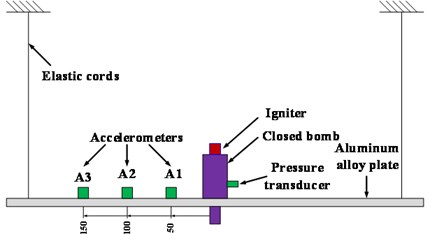a)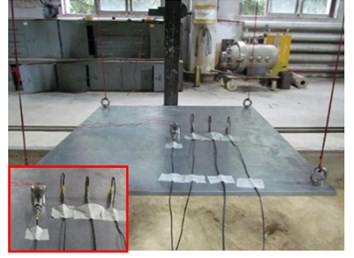b)

During the tests, the pyroshock signals were recorded by piezoelectric accelerometers of the range ±50,000 g, as shown in Fig. 1(a). Three measurement points were located on the upper surface of the plate, respectively 50 mm,100 mm and 150 mm away from the center. Piezoelectric accelerometers, the pressure transducer, and the sensor conditioner were used with a DAQ system. The sampling rate and the sampling time were set to 1 MHz and 20 ms respectively. The eight-pole Butterworth bandpass filter between 100 Hz to 30 kHz was applied for accelerometers results to resolve possible issues such as low frequency data drift and data aliasing during data processing .

Pyroshock measurement tests were performed 3 times under different amounts of explosive to improve the credibility of the test data. An abnormal data was obtained for 200 mg due to insufficient combustion of explosive and a test was performed again. The results of tests are discussed in next section.

## 3. Results and discussion

### 3.1. Effect of amount of explosive on output pressure

After the detonation of the igniters, the pressure inside the closed bomb reaches peak value and then the pressure value gradually decreases. The peak pressure values under different amount of explosive are summarized in Table 1.

Table 1Peak pressure results

 Amount of explosive (mg) Test1 (MPa) Test2 (MPa) Test3 (MPa) Average (MPa) 50 27.07 18.92 17.88 21.29 100 35.41 34.14 30.56 33.37 150 49.38 54.81 41.07 48.42 200 64.37 73.63 73.61 70.53

Fig. 5Effect of amount of explosive on output pressure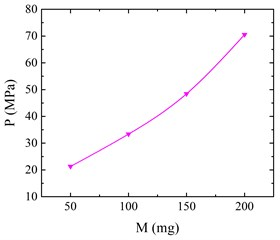From the Fig. 5, it is clearly that the peak pressure values increase along with increased amount of explosive. Using the data, the Nobel-Abel equation of state  can be improved to estimate the explosive force of the main charge of the igniter:

1
${P}_{m}={P}_{0}+\frac{fM}{{V}_{0}-\alpha M},$

where $f$ is the explosive force (J/g), ${P}_{m}$ is the peak pressure (MPa), ${V}_{0}$ is the volume of the closed bomb (2.5 ml); $M$ is the charge of the igniter (g); $\alpha$ is the co-volume of explosive (cm-3/g). ${P}_{0}$ is the equivalent output pressure of the primers.

The relationship between the peak output pressure and the amount of explosive is in accordance with the Nobel-Abel equation of state. It is estimated that the explosive force $f$ and co-volume $\alpha$ of main explosive are respectively 402.1 J/g and 5.58 cm-3/g. The equivalent output pressure of the primers ${P}_{0}$ is 12.37 MPa.

### 3.2. Effect of amount of explosive on pyroshock

The maximax shock response spectra (SRS) are calculated from 100 Hz to 10 kHz with a damping ratio 5 % ($Q=$10). The typical measured acceleration data is showed in Fig. 6(a), while Fig. 6(b) presents the corresponding calculated SRS.

Fig. 6Pyroshock results at point1 under 50 mg explosive: a) acceleration data, b) SRS calculated from acceleration data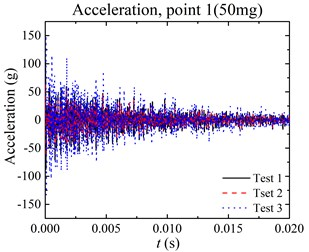a)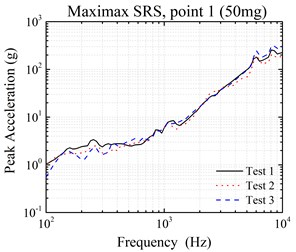b)

Fig. 7 shows the variation of peak acceleration with different locations. For the same amount of explosive, the intensity of peak acceleration reduces as it is away from the pyroshock source. In particular, an igniter with larger amounts of explosive has higher pyroshock level at the same measured point.

From the experiment, it is found that the peak acceleration response will increase with the increased amount of explosive. Fig. 8 shows that acceleration trends of point 2 and point 3 are almost same, except for the point 1. The gradient of the curve is less for point 2 and point 3 while it is steep for point1. It shows that material stress wave propagation effects govern the response for point 1. However, pyroshock response is governed by the combination of material stress wave propagation and structural resonance response effects for point 2 and point 3 .

Fig. 7Effect of locations on peak acceleration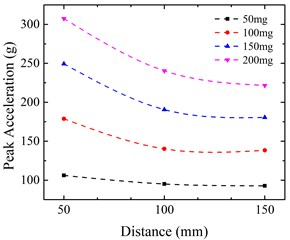Fig. 8Effect of amount of explosive on peak acceleration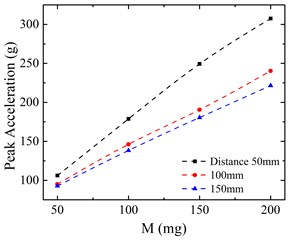The SRS of different amounts of explosive are compared and analyzed for different locations as depicted in Fig. 9. Due to structural resonance response effects, the amount of explosive has smaller effects on SRS at low frequency (< 1000 Hz) compared with SRS at high frequency (> 1000 Hz), especially for point 2 and poin 3. To get a quantitative expression of the relation, dimensionless coefficients without regard to frequency are defined as:

2

where, the $A$ is the mean relative coefficient of SRS, the $M$ is the relative coefficient of max SRS. $SR{S}_{1}$ is the base SRS. In this study, the SRS of 50 mg is the base one.

Fig. 9SRS comparison: a) SRS at point1, b) SRS at point2, c) SRS at point3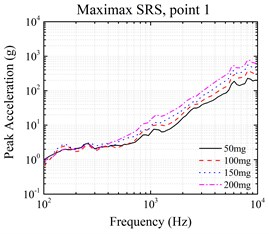a)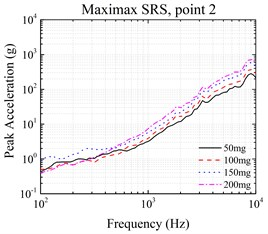b)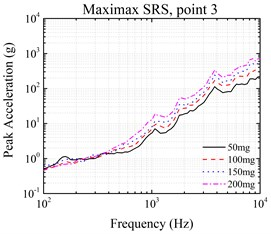c)

The $A$ and $M$ under different amount of explosive are shown in Fig. 10, as well as their linear fit. Increases of the $A$ and $M$ are observed when the amount of explosive increased. The linear correlation coefficients of the $A$ and $M$ are respectively 0.98 and 0.99. Both coefficients show an approximately linear relation between the explosive amount and the SRS coefficients.

Fig. 10A and M of different amount of explosive: a) A, b) M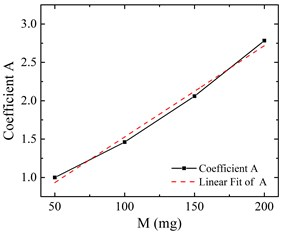a)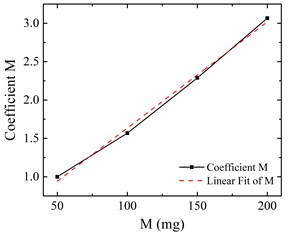b)

## 4. Conclusions

In this study, effects of modification of amount of explosive on generated pyroshock and pressure of igniters are analyzed using experimental method. The characteristics of output pressure, acceleration response and SRS under different amount of explosive are studied. The relation between the amount of explosive and peak pressure shows good agreement with Nobel-Abel equation of state (EOS). It is found that pyroshock shows an approximate linear growth with increased amount of explosive both in peak acceleration and SRS.